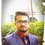# Q.1

     Take as input a natural number, say n. Output all subsets of {1,2,...,n}.
You cannot use functions or recursion.


# Q.2

     Write a program to read a sequence of non-zero integers till the number zero is entered and at the end display the following:
a)      the number of even and odd numbers,
b)      sum of all the numbers entered,
c)      the length and starting index (or position) of a largest subsequence of consecutive non-decreasing integers entered. Assume that the index of the numbers start from 1.

For example, if the user input is  1 -2 3 17 9 5 -10 -12 0, then the output will be:
No. of even numbers: 3
No. of odd numbers: 5
Sum of the numbers: 11
Length of largest non-decreasing subsequence: 3
Starting index: 2
Note: Do not use arrays.Note by Rishabh Deep Singh
4 years, 11 months ago

This discussion board is a place to discuss our Daily Challenges and the math and science related to those challenges. Explanations are more than just a solution — they should explain the steps and thinking strategies that you used to obtain the solution. Comments should further the discussion of math and science.

When posting on Brilliant:

• Use the emojis to react to an explanation, whether you're congratulating a job well done , or just really confused .
• Ask specific questions about the challenge or the steps in somebody's explanation. Well-posed questions can add a lot to the discussion, but posting "I don't understand!" doesn't help anyone.
• Try to contribute something new to the discussion, whether it is an extension, generalization or other idea related to the challenge.

MarkdownAppears as
*italics* or _italics_ italics
**bold** or __bold__ bold
- bulleted- list
• bulleted
• list
1. numbered2. list
1. numbered
2. list
Note: you must add a full line of space before and after lists for them to show up correctly
paragraph 1paragraph 2

paragraph 1

paragraph 2

[example link](https://brilliant.org)example link
> This is a quote
This is a quote
    # I indented these lines
# 4 spaces, and now they show
# up as a code block.

print "hello world"
# I indented these lines
# 4 spaces, and now they show
# up as a code block.

print "hello world"
MathAppears as
Remember to wrap math in $$ ... $$ or $ ... $ to ensure proper formatting.
2 \times 3 $2 \times 3$
2^{34} $2^{34}$
a_{i-1} $a_{i-1}$
\frac{2}{3} $\frac{2}{3}$
\sqrt{2} $\sqrt{2}$
\sum_{i=1}^3 $\sum_{i=1}^3$
\sin \theta $\sin \theta$
\boxed{123} $\boxed{123}$

Sort by:

  1 2 3 4 5 6 7 8 9 10 11 12 13 14 15 16 17 18 19 20 21 22 23 24 25 26 27 28 29 30 31 32 33 34 35 36 37 38 39 40 41 42 43 #include int main() { int n=1, e=0, o=0, sum=0, p=0, i=0, index=1, c=0, gre=0, first=1; printf("Enter a sequnce of numbers terminal no. of sequence being 0"); while(n!=0) { i++; scanf("%d" ,&n); if(n==0) break; sum+=n; if(n%2==0) e++; else o++; if(n>=p) { c++; } else { if(c>gre) { gre=c; first=index; index=1; } c=1; }p=n; } if(c>gre) { gre=c; first=index; } printf("No. of even no. is %d \n" ,e); printf("No. of odd numbers is %d \n" ,o); printf("Sum of the entered numbers is %d \n" ,sum); printf("Length of largest non decreasing subsequence within is %d \n" ,gre); printf("starting index is %d \n" ,first); return 0; } 

- 4 years, 11 months ago

I have increased the scope of your problems from C to all other languages by editing "C" out of the discussion.

Hint about 2: How do functions work? What is the underlying data structure that keeps track of where the execution should return to?

- 4 years, 11 months ago

Thanks @Agnishom Chattopadhyay Can u post a solution to Question no 1.

- 4 years, 11 months ago

- 4 years, 11 months ago

i need to submit my assignment now please post the answer Bro.

- 4 years, 11 months ago

  1 2 3 4 5 6 7 8 9 10 11 12 13 14 15 16 17 18 19 20 21 22 23 24 25 26 27 28 29 30 31 32 33 34 35 #include #include int main() { int n,i,r=1,z,b=0,rem,k=0,j=1; printf("Enter the number\n"); scanf("%d",&n); for(i=0;i

- 4 years, 11 months ago

- 4 years, 11 months ago

Nice!

- 4 years, 11 months ago

Thanks

- 4 years, 11 months ago